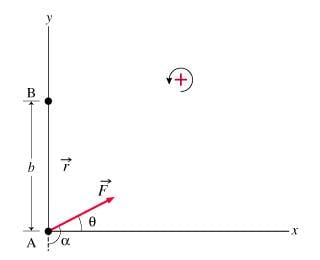# Finding torque

## Homework Statement

What is the torque τB about axis B due to the force F⃗ ? (B is the point at Cartesian coordinates (0,b), located a distance b from the origin along the y axis.)
Express the torque about axis B in terms of F, θ, ϕ, π, and/or other given coordinate data.[/B]
τ = RF sin (θ)

## The Attempt at a Solution

So I know that the answer is Tb = bFsin (pi/2+θ)
What I don't understand is how to get pi/2+θ?
I know that θ is the angle between r vector and F vector and since that's the case shouldn't the answer be pi/2-θ?

#### Attachments

Orodruin
Staff Emeritus
Homework Helper
Gold Member
Can you please show us your own effort? What did you get?

Can you please show us your own effort? What did you get?
Well I thought the answer was Tb = bFsin (pi/2-θ) but that's obviously wrong. I would like to know why. My homework says that alpha is the angle between r vector and F vector therefore I thought that it would be pi/2-θ.

Orodruin
Staff Emeritus
$$\sin(\pi - \alpha) = \sin(\pi)\cos(\alpha) - \cos(\pi)\sin(\alpha) = 0 + \sin(\alpha) = \sin(\alpha).$$
$$\sin(\pi/2 - \theta) = \sin(\pi/2)\cos(\theta) - \sin(\theta)\cos(\pi/2) = \cos(\theta)\sin(\pi/2) = \cos(\theta)\sin(\pi/2) + \sin(\theta)\cos(\pi/2) = \sin(\pi/2 + \theta).$$